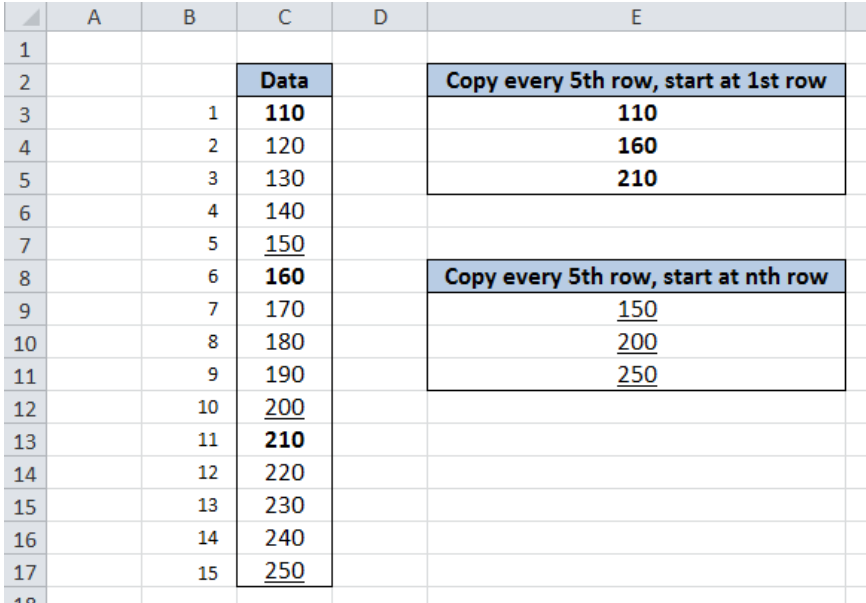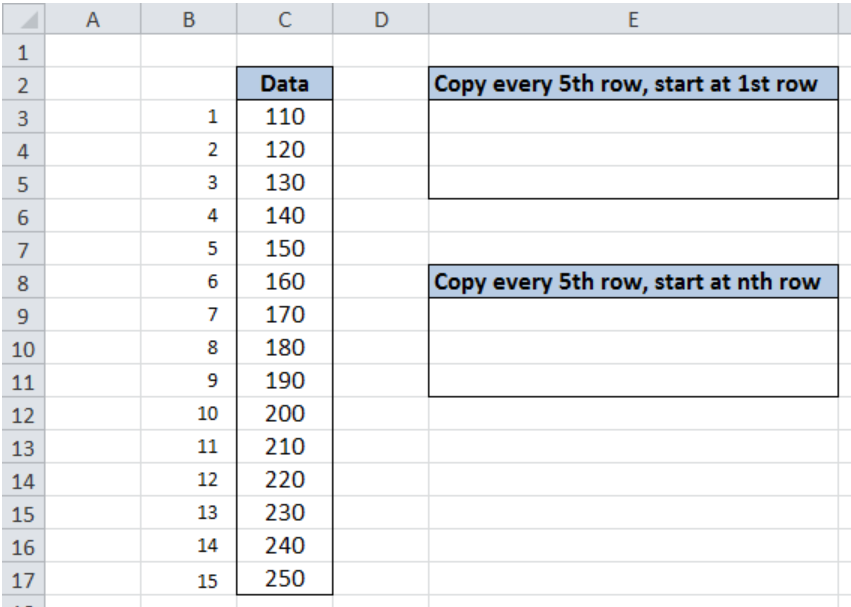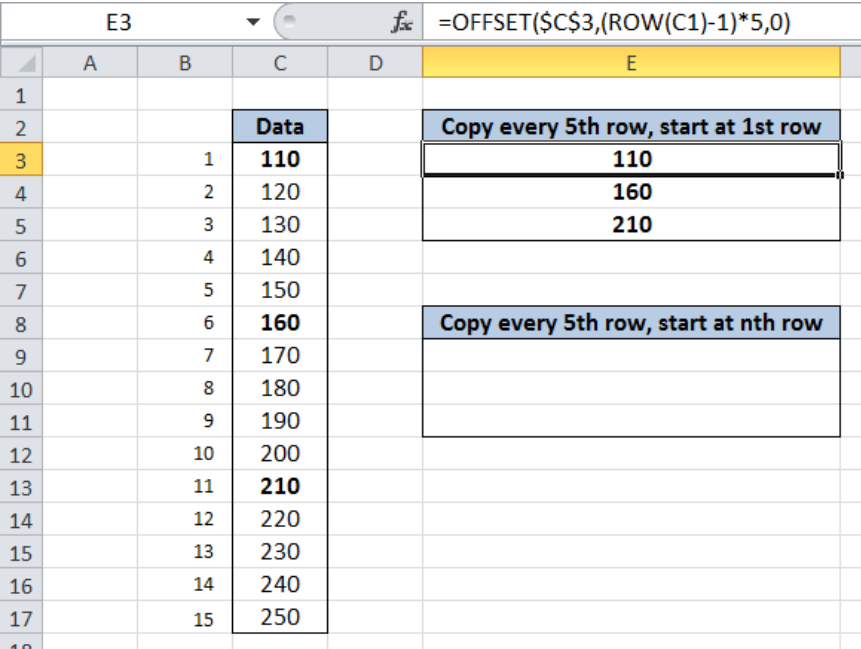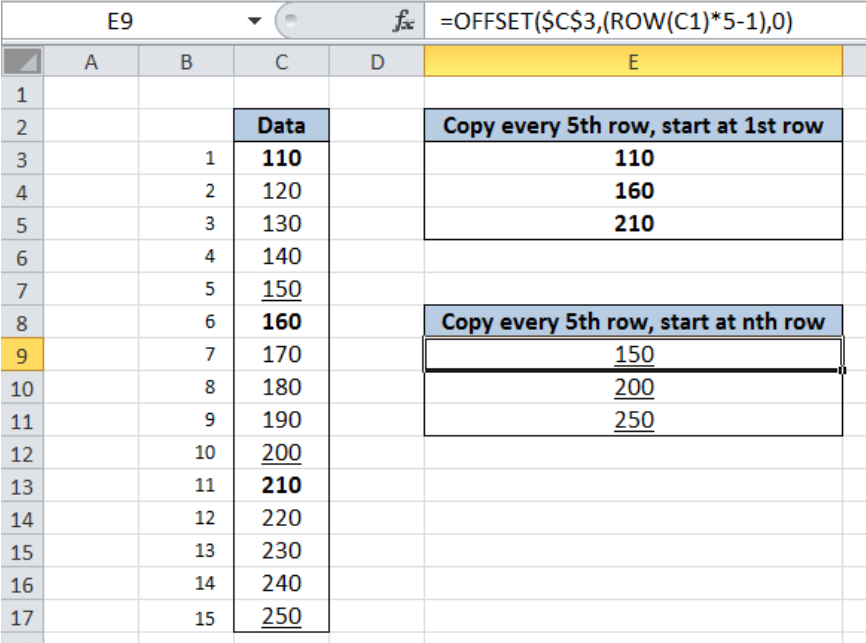Get instant live expert help with Excel or Google Sheets“My Excelchat expert helped me in less than 20 minutes, saving me what would have been 5 hours of work!”

#### Post your problem and you’ll get expert help in seconds.

Your message must be at least 40 characters
Our professional experts are available now. Your privacy is guaranteed.

# Copy Value from Every nth Row

If you want to copy every nth row in Excel, you can accomplish this by combining several functions.

## Copy a value from every nth row in Excel

Copying values with a specific pattern in Excel can be done using the OFFSET and ROW functions. OFFSET function returns a reference to a cell or range while ROW function returns the row number of a cell.  This step by step tutorial will assist all levels of Excel users in copying values from every nth row.Figure 1. Final result: Copy value from every nth row

Final formula starting at 1st row : `=OFFSET(\$C\$3,(ROW(C1)-1)*5,0)`

Final formula starting at nth row : `=OFFSET(\$C\$3,ROW(C1)*5-1,0)`

### Syntax of the OFFSET function

`=OFFSET(reference, rows, cols, [height], [width])`

The parameters of OFFSET are:

• reference – The reference cell where we will base the offset
• rows – The number of rows either up or down the reference cell that determines the offset
• Cols – The number of columns either left or right of the reference cell that determines the offset
• Height and width are both optional; Height determines the number of rows while width determines the number of columns that we want the returned reference to be

### Syntax of the ROW function

`=ROW(reference)`

• reference – The cell or range of cells whose row number we want to determine

### Setting up Our Data

Our data in column C shows a list of fifteen numbers, which are numbered accordingly for easy reference.  In cells E3:E5, we will obtain the values every 5th row starting with the first value, while in cells E9:E11, we will obtain the values every 5th row starting with the 5th value.Figure 2. Sample data to copy values from every nth row

### Copy Value from Every nth Row

The formula to copy the values from every nth row depends on the first value that we want to copy.  We could start at the first row or the nth row.

Formula starting with 1st row: `=OFFSET(first_cell,(ROW(C1)-1)*n,0)`

Formula starting with nth row: `=OFFSET(first_cell,(ROW(C1)*n-1),0)`

The first cell in our data serves as the reference cell for the OFFSET function.

### Copy every 5th row, starting with 1st rowFigure 3. Formula to copy values from every 5th row, starting with 1st row

To copy values from every 5th row, starting with the first row in our data, we follow these steps:

Step 1.  Select cell E3.

Step 2.  Enter the formula: `=OFFSET(\$C\$3,(ROW(C1)-1)*5,0)`

Step 3.  Press ENTER

Step 4.  Copy and paste the formula to the succeeding cells E4 and E5

The reference used is the first cell in the list which is C3.  The ROW formula “`(ROW(C1)-1)*5`” determines the offset for the rows, which returns a multiple of 5.

In cells E3, E4 and E5, the 1st, 6th, and 11th values are copied.  Finally, the results are 110, 160 and 210.

### Copy every 5th row, starting with 5th rowFigure 4. Copy values from every 5th row, starting with the 5th row

To copy values from every 5th row, starting with the fifth row in our data, we follow these steps:

Step 1.  Select cell E9.

Step 2.  Enter the formula: `=OFFSET(\$C\$3,(ROW(C1)*5-1),0)`

Step 3.  Press ENTER

Step 4.  Copy and paste the formula to the succeeding cells E10 and E11

The ROW formula “(ROW(C1)*5-1)” determines the offset for the rows, which returns the values 4, 9 and 14.  This enables our OFFSET formula to return the cells corresponding to the 5th, 10th, and 15th rows.

In cells E9, E10 and E11, the 5th, 10th, and 15th values are copied.  The results are 150, 200 and 250.

Most of the time, the problem you will need to solve will be more complex than a simple application of a formula or function. If you want to save hours of research and frustration, try our live Excelchat service! Our Excel Experts are available 24/7 to answer any Excel question you may have. We guarantee a connection within 30 seconds and a customized solution within 20 minutes.

### Did this post not answer your question? Get a solution from connecting with the expert.Another blog reader asked this question today on Excelchat:
Related blogs
Solution examplesI need help fixing a Formula on Sheet 2, starting in Column G Row 22. In short, the formula should average the last six dollar amounts unless one of the last 6 dollar amounts is blank or 0. G56 on the same sheet works with the same logic but for only 3 months.
Solved by B. L. in 41 minsHello! There is a column with a certain number of rows in it. I need to calculate two averages: the average of every second row starting from the 1st one (aka the average of every odd-th cell) and the average of every second row starting from the 2nd one (aka the average of every even-th cell). I got tangled up with =OFFSET... Thanks!
Solved by C. J. in 37 minsin range b2:o2, I am trying to write a formula to count if the values are equal to 1 thru 27.
Solved by E. L. in 52 minsRepost I need a formula written into cells (AI8 to EK8) to identify patterns and then count specific data. sheet name: SHOPEE PATTERNS ONE ROW have data of different shooter (wins and losses from cells B to H) that I need identify. There were 2 rows (AI4:EK4 and AI2:EK2) that will determine which cells to count and which to keep blank after applying the formula.
Solved by S. H. in 9 minsI need a formula written into cells (AI8 to EK8) to identify patterns and then count specific data. sheet name: SHOPEE PATTERNS ONE ROW have data of different shooter (wins and losses from cells B to H) that I need identify. There were 2 rows (AI4:EK4 and AI2:EK2) that will determine which cells to count and which to keep blank after applying the formula.
Solved by E. S. in 60 mins## Subscribe to Excelchat.coAnother blog reader asked this question today on Excelchat: